# ML Aggarwal Class 7 Solutions for ICSE Maths Chapter 9 Linear Equations and Inequalities Ex 9.2

## ML Aggarwal Class 7 Solutions for ICSE Maths Chapter 9 Linear Equations and Inequalities Ex 9.2

Question 1.
If 7 is added to five times a number, the result is 57. Find the number.
Solution:Question 2.
Find a number, such that one-fourth of the number is 3 more than 7.
Solution:Question 3.
A number is as much greater than 15 as it is less than 51. Find the number.
Solution:Question 4.
If $$\frac { 1 }{ 2 }$$ is subtracted from a number and the difference is multiplied by 4, the result is 5. What is the number?
Solution: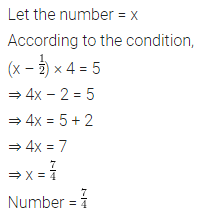Question 5.
The sum of two numbers is 80 and the greater number exceeds twice the smaller by 11. Find the numbers.
Solution:Question 6.
Find three consecutive odd natural numbers whose sum is 87.
Solution: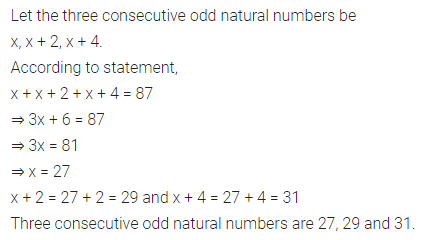Question 7.
In a class of 35 students, the number of girls is two-fifths of the number of boys. Find the number of girls in the class.
Solution: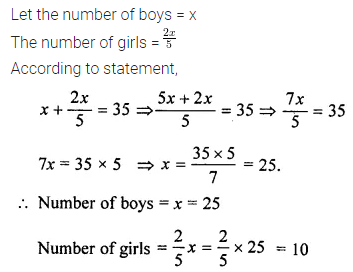Question 8.
A chair costs ₹ 250 and the table costs ₹ 400. If a housewife purchased a certain number of chairs and two tables for ₹ 2800, find the number of chairs she purchased.
Solution:Question 9.
Aparna got ₹ 27840 as her monthly salary and over-time. Her salary exceeds the overtime by ₹ 16560. What is her monthly salary?
Solution: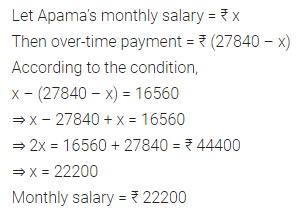Question 10.
Heena has only ₹ 2 and ₹ 5 coins in her purse. If in all she has 80 coins in her purse amounting to ₹ 232, find the number of ₹ 5 coins.
Solution:Question 11.
A purse contains ₹ 550 in notes of denominations of ₹ 10 and ₹ 50. If the number of ₹ 50 notes is one less than that of ₹ 10 notes, then find the number of ₹ 50 notes.
Solution: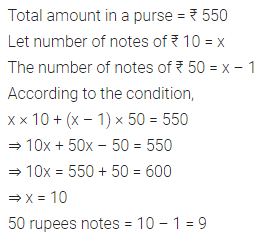Question 12.
After 12 years, 1 shall be 3 times as old as I was 4 years ago. Find my present age.
Solution: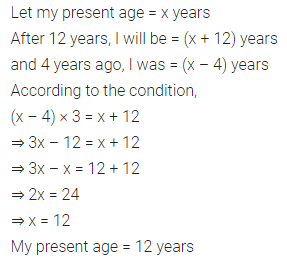Question 13.
Two equal sides of an isosceles triangle are 3x – 1 and 2x + 2. The third side is 2x units. Find x and the perimeter of the triangle.
Solution:Question 14.
The length of a rectangle plot is 6 m less than thrice its breadth. Find the dimensions of the plot if its perimeter is 148 m.
Solution:Question 15.
Two complementary angles differ by 20°. Find the measure of each angle.
Solution: